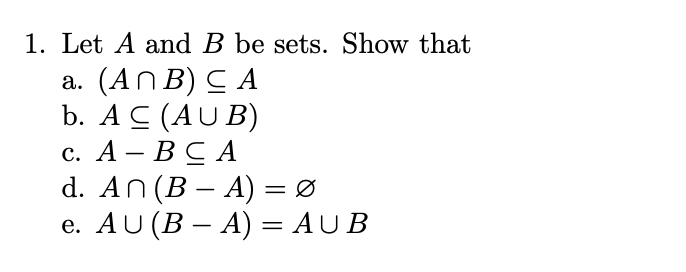# Question 3 Part III 1. Let A and B be sets. Show that a. (A ∩ B) ⊆ A b. A ⊆ (A ∪ B) c. A − B ⊆ A d. A ∩ (B − A) = ∅ e. A ∪ (B − A) = A ∪ B 1. Let A and B be sets. Show that a. (ANB) CA b. A C (AUB) C. A - B CA d. An (B – A) = 0 e. AU (B – A) = AUB